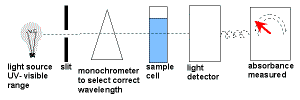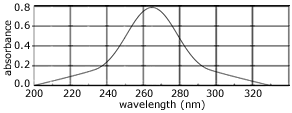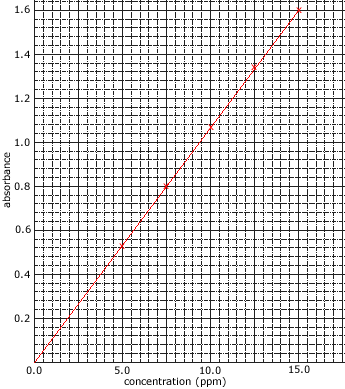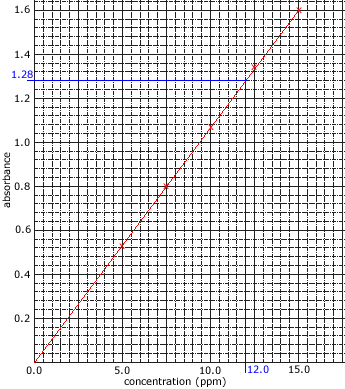UV-Visible Spectroscopy Chemistry Tutorial

Key Concepts

• Some compounds absorb light with wavelengths in the ultraviolet and/or visible range of the electromagnetic spectrum (200 nm to 780 nm).
Valence shell electrons, either bonding or non-bonding electrons, most easily absorb this energy.
The electron in the ground state (lower energy level) absorbs this energy and moves to a higher energy level (excited state).• UV-Visible spectroscopy uses a UV-visible spectrometer.
White light enters a monochromator which narrows down the wavelength band of the radiation.
This monochromatic light is split into two beams.
One beam, the reference beam, passes directly to the detector to measure its intensity.
The other beam passes through the sample which absorbs some of the light before it enters the detector where its intensity is measured.
The intensity of the reference beam is compared to the intensity of the other beam at each wavelength in order to give a measurement of the sample's absorption.
• The amount of light absorbed is proportional to the concentration of the compound in solution.
Concentrations are often expressed as mg L-1 or ppm.
• The amount of light absorbed by the sample is compared to the amount of light absorbed by a set of standards of known concentration.

No ads = no money for us = no free stuff for you!

Example

Toluene is an important industrial chemical.
The concentration of toluene in a solution can be determined using UV-visible spectroscopy.

1. Choose an appropriate wavelength: a wavelength at which there is significant absorbance .The UV-visible spectrum of a solution of 7.5 ppm toluene is shown on the right.
It can be seen that the maximum absorbance of toluene occurs at 265 nm.
265 nm is the best wavelength to choose in order to determine the concentration of toluene in solution.

2. Prepare a set of standard solutions of toluene.
A standard solution is a solution with a known concentration.

3. Measure the absorbance of each standard solution at the selected wavelength (265 nm).
It is usually best to measure the absorbance of each solution several times and then take the average absorbance to reduce errors.
Example:
concentration
(ppm)
average
absorbance
0.0 0.0
5.0 0.5
7.5 0.8
10.0 1.1
12.5 1.3
15.0 1.6

4. Plot the calibration graph.5. Measure the absorbance, at the same wavelength, of the sample solution of unknown concentration.
At 265 nm the solution of unknown toluene concentration had an absorbance of 1.28

6. Plot the absorbance of the sample solution on the calibration graph and read off the concentration.The concentration of toluene in the sample solution is 12.0 ppm.

Can you apply this?

Join AUS-e-TUTE!

Take the exam now!